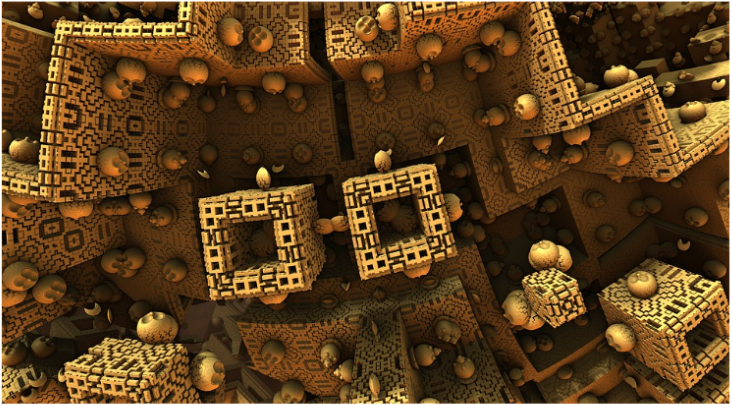# Applications of Calculus: Rendering 3D Computer Graphics

Introducing Kajiya's rendering equation.

This post is part of a series.By including lighting and shadows, 2D computer graphics can be made to appear 3D. The process by which this is done is called rendering, and it makes use of calculus to simulate the light that bounces around in a scene as it makes its way to the camera.

Suppose we are looking at point $x$ from angle $\theta_0.$ We see light coming from sources (e.g. sun, overhead lights) which we will call $L_{direct}(x, \theta_0),$ and there is also light that hits the point from other angles $\theta$ after bouncing around. We will call this bounced light $L_{bounce}(x, \theta).$ However, only a proportion of bounced light that hits the point will reflect to our viewing angle $\theta_0.$ We will call this proportion $p(x, \theta, \theta_0).$

The total light coming to us when we look at point x from angle $\theta_0$ is calculated by adding up all the light arriving from sources (like the sun, or overhead lights) as well as the light reflected after bouncing off other surfaces in the scene. To sum up all the light reflected to us after bouncing off other surfaces, we have to integrate over the hemisphere.

Thus, we calculate the total light we see at point $x$ from angle $\theta_0$ using the following formula:

\begin{align*} L_{total}(x, \theta_0) = L_{direct}(x, \theta_0) + \int p(x, \theta, \theta_0) L_{bounce}(x, \theta) \cos (\theta) d \theta \end{align*}

This integral is called Kajiya’s Rendering Equation, and it is often approximated in computer graphics to compute the total light coming from a point when viewed from a particular angle.

This post is part of a series.

Tags: